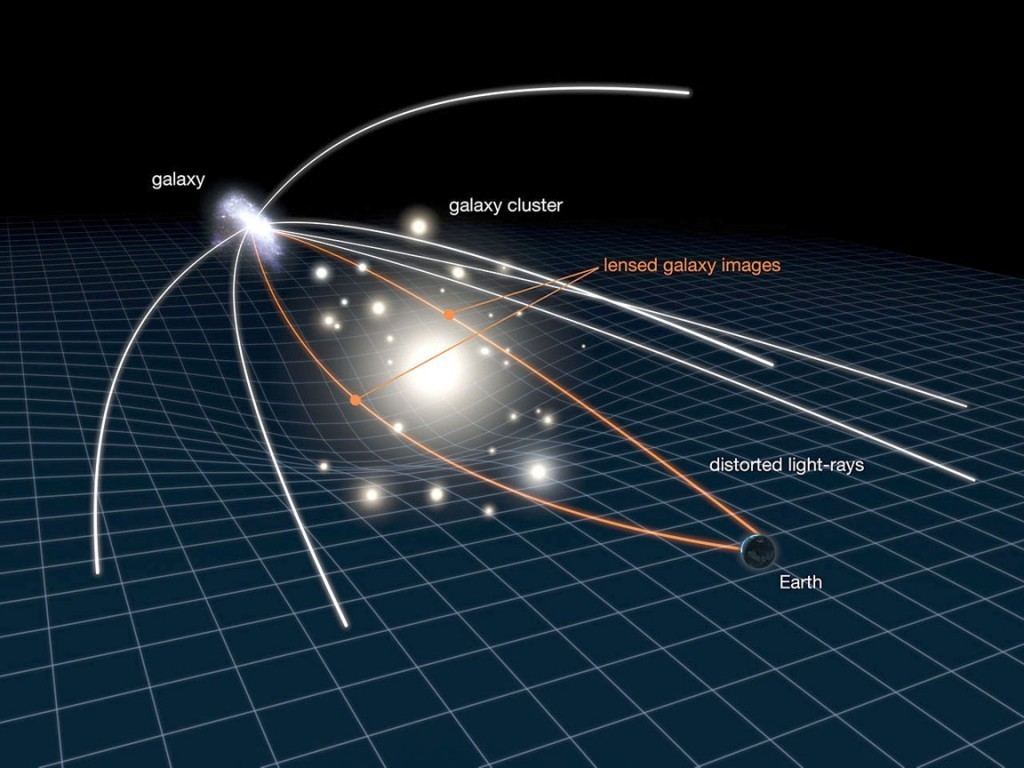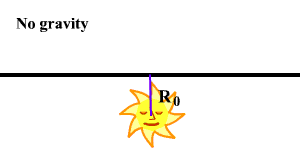# Bend It Like Newton

In Gravity by Brian Koberlein16 Comments

Yesterday’s post on testing the assumption that photons are massless raised a few questions for readers. One of the most common was the idea that the gravitational lensing of light must mean that photons have mass. After all, if a star or galaxy can deflect light gravitationally, doesn’t that mean the light is gravitationally attracted to it? If that is the case, doesn’t that mean that light has mass?

Before we delve into the question, we first need to be clear about what we mean by “mass”. There are actually several different types of mass. The type that best corresponds to our intuitive understanding is known as inertial mass. Inertial mass is determined by its resistance to acceleration. If you push on objects with a force, an object with less inertial mass will accelerate more than one with more inertial mass.

Another type of mass is known as gravitational mass. Gravitational mass is what (in Newton’s gravity) causes the gravitational attraction between objects. When you step on a scale in the morning, you are measuring your gravitational mass. While technically gravitational mass and inertial mass are not the same thing, we generally treat them as the same thing because of the “principle of equivalence”.

If you release a ball from the leaning tower of Pisa, the gravitational force on the ball causes it to fall. The strength of that force depends on the gravitational mass of the ball, but the rate at which it falls depends on the inertial mass of the ball. But experiments have shown that masses all fall at the same rate in a gravitational field, so that means the gravitational and inertial masses must have the same value. This equality between gravitational and inertial mass is called the principle of equivalence. While this was known since at least Galileo’s time, it was Einstein who made the idea central to our understanding of gravity.

The third type of mass is known as relativistic mass. This stems from Einstein’s theory of special relativity and the equivalence of mass and energy (the famous E equals m c squared). In that famous equation, E is the energy of a particle, and c is the speed of light. So if you divide the energy of a particle by the speed of light squared, you get a “mass”, known as the relativistic mass of the particle.

Now if an object is at rest (relative to you) then the relativistic mass has the same value as the inertial mass. This is sometimes called the “rest mass” of an object. But in general, relativistic mass is not the same thing as inertial or gravitational mass. Unfortunately this point isn’t often made clear, so it leads to a great deal of confusion. When someone says “the mass of an object increases as it approaches the speed of light”, that’s really the relativistic mass. A fast moving object has not only energy due to its rest/inertial mass, but also a kinetic energy due to its motion. The relativistic mass due to its total energy is what increases. Its inertial (and gravitational) mass is unchanged.

This is the key difference. Relativistic mass is an apparent mass that depends on how the object is moving relative to you. Inertial and gravitational mass are inherent properties of an object, and don’t depend on your point of view.

So what does this have to do with whether photons have mass? Photons have energy, so we can define the relativistic mass of a photon by taking its energy and dividing by the speed of light squared. The energy of a photon depends upon its wavelength. Long wavelength (reddish light) photons have less energy than short wavelength (bluish light) photons. This means photons have different relativistic masses.

Photons don’t have “rest mass” or inertial mass. Despite popular news articles about “stopping light”, you can’t hold a photon in place. The “light stopping” experiments are effects of light waves, which is a whole other rabbit hole. You also can’t accelerate light with a force. The speed of a photon is constant, so again, no inertial mass. By the equivalence principle, that also means they have no gravitational mass.

At least that is the accepted answer. Maybe for photons, their relativistic mass is their inertial/gravitational mass. How do we know it’s not? Actually, we have an experiment that proves it, and Arthur Eddington first did it in 1919.

In 1919 Eddington photographed the positions of stars near the Sun during a total eclipse. He compared those positions to their positions when the Sun wasn’t there, and found that they had appeared to shift away from the sun. This is because the Sun gravitationally deflected the starlight slightly. This bending of light made the stars appear to be in a different direction. Einstein predicted this light bending due to the curvature of space in his theory of general relativity. Thus, Eddington proved that Einstein’s theory was correct.When this story is presented, it’s often said that since photons have no mass Newton’s model predicts light shouldn’t bend. Einstein’s theory predicts light bending, so this proved Einstein right. But actually that isn’t entirely the case. If the relativistic mass of a photon is equated to its inertial and gravitational mass, then Newton’s gravity does predict light bending.

The catch is that the amount of bending predicted by Newton’s model is half what Einstein’s model predicted. Eddington actually demonstrated not only that light was gravitationally deflected, but that the amount matched Einstein, and not Newton. You can see this in the figure above, which shows three possible outcomes for light pending: Newton is right, and photons are massless (no deflection), Newton is right and photons have mass (some deflection), or Einstein is right, photons are massless and space is curved (more deflection).

So the gravitational lensing we see from stars and galaxies actually demonstrates that photons aren’t being gravitationally attracted in the Newtonian sense. Instead, space is warped by mass of stars and galaxies, and the path of light is warped accordingly. Light really is massless. You can’t bend it like Newton, but you can bend it like Einstein.

1.” the gravitational force on the ball causes it to fall. The strength of that force depends on the gravitational mass of the ball,”

hummrm… I always thought that the strength of the force depended on the gravitational mass of the *Earth*, not of the ball… the gravitational mass of the ball would determine the (very tiny, for a “normal” ball) strength of the force pulling the Earth *up*… so, was I wrong?

1.Author

It actually is both. Fun fact, when you stand on a scale to weigh yourself, you measure not only your weight in the Earth’s gravitational field, but the Earth’s weight in your gravitational field.

2.Your correct, the mass of the ball in relation to the mass of the Earth is really insignificant..Therefore all objects fall at the same rate..

3.The force that a ball applies to the Earth is the same as the Earth applies to the ball. This follows from Newton’s third law. But because the Earth is so heavy (inertial mass) this does not matter much. But for the ball it does 😉 So that’s why the ball falls to the Earth and not the other way around, even though the size of the force on both objects is equally big.

2.“three possible outcomes for light pending”

I think you meant *bending*, not “pending”.

1.Author
3.Regarding my first comment above, I thought a little more about it and I think I get it now: the Newtonian *force* between ball and Earth varies accordingly to the product of the (gravitational) masses of both ball and Earth, as you wrote. What remains constant regardless of the ball’s mass is the *acceleration* on the ball, because it is equal to that force above divided by the ball’s (inertial) mass. This happens because, as inertial mass equals gravitational mass, the ball’s mass cancels itself out, and that is why any ball’s (in fact, any body’s) acceleration is the same in the Earth’s gravitational field, regardless of its mass…

Thanks for another great, thought-provoking article!

4.As you said, the energy of a photon depends on its wavelength. Also, relativistic mass depends on the energy. As a consequence, photons with a longer wavelength should get deflected lesser than photons with a shorter wavelength. Hence, when we see gravitational lensing, we should also see dispersion of the light, just like a prism. Have we actually observed this dispersion? If we haven’t, what’s the reason?

1.Author

In general relativity, the bending of light doesn’t depend upon wavelength, so you don’t get that kind of dispersion.

1.Thanks for the clarification, Brian!

1.Your question segues nicely into another post from Prof Koberlein:
https://briankoberlein.com/2015/03/26/over-the-rainbow/

Rainbow gravity is a new idea, so Prof responsibly takes it with heavy salt. But if true, then you would see your “dispersion of light” from gravitational lensing. It just requires much more mass than a star… say, a black hole.

5.Thanks for this good clarification on much ill-quoted Eddington’s finding.

Off topic question. Feynman has fooled me to think that interaction between two electrons may happen, but with lower probability, when faster or slower than light is needed. Is this true?

6.The experiment in 1919 and the further experiments in the mid 60’s by Shapiro, prove a star/Sun distorts time space, but to conclude this curves space is a leap of faith.
Shapiro values of this time space shift were extremely small, and after only a small incremental increase in distance from the Sun they totally disappear..
How would this prove Einstein’s field of curved space and demonstrate gravity?
With so small of a values how would this equate to the gravity required for the Solar system?
With no time space altered with just a small distance increase from the Sun, How does this explain the gravity of the solar system..??
Einsteins theory’s are non linear math explanation that give approximate answers of an observed phenomenon.not an observed empirical solution.
It’s time to move on…and I doubt a western world University will move science forward..new thoughts are required..maybe from eastern/ Asian Universities will find a real solution to time space.

1.Author

The 1919 Eddington experiment and the Shapiro work of the 1960s were only the first observations of their kind. Since then we’ve made extremely precise observations of general relativity, which has confirmed it to an extremely high level of precision. Relativity is a robust scientific theory, and to argue that it isn’t is simply ignoring reality.

7.Newton also predicting bending of light, based on a tiny mass for photons. Supposedly his prediction was not as accurate as Einstein’s. Einstein’s original prediction was the same as Newton’s angle of deflection. Maybe Newton just needed a different mass for photons to get the same bending as observed?

8.Good stuff which clarifies the specific point of the equivalence principle very well, when confronted with question that if a photon has energy and therefore mass why should we believe in space-time curvature; surely Newton was correct after all.